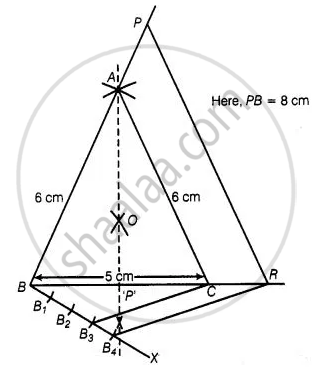# Draw an isosceles triangle ABC in which AB = AC = 6 cm and BC = 5 cm. Construct a triangle PQR similar to ∆ABC in which PQ = 8 cm. Also justify the construction. - Mathematics

Diagram
Sum

Draw an isosceles triangle ABC in which AB = AC = 6 cm and BC = 5 cm. Construct a triangle PQR similar to ∆ABC in which PQ = 8 cm. Also justify the construction.

#### Solution

Let ΔPQR and ΔABC are similar triangles, then its scale factor between the corresponding sides is (PQ)/(AB) = 8/6 = 4/3

Steps of construction:

1. Draw a line segment BC = 5 cm.

2. Construct OQ the perpendicular bisector of line segment BC meeting BC at P’.

3. Taking B and C as centres draw two arcs of equal radius 6 cm intersecting each other at A

4. Join BA and CA. So, ΔABC is the required isosceles triangle.5. From B, draw any ray BX making an acute ∠CBX

6. Locate four points B1, B2, B3 and B4 on BX such that BB1 = B1B2 = B2B3 = B3B4

7. Join B3C and from B4 draw a line B4R || B3C intersecting the extended line segment BC at R.

8. From point R, draw RP||CA meeting BA produced at P
Then, ΔPBR is the required triangle.

Justification:

∵ B4R || B3

∴ (BC) - (CR) = 3/1

Now, (BR)/(BC) = (BC + CR)/(BC)

= 1 + (CR)/(BC) = 1 + 1/3 = 4/3

Also, RP || CA

∴ ΔABC ∼ ΔPBR

And (PB)/(AB) = (RP)/(CA) = (BR)/(BC) = 4/3

Hence, the new triangle is similar to the given whose sides are 4/3 times of the corresponding sides of the isosceles ΔABC.

Concept: Division of a Line Segment
Is there an error in this question or solution?

#### APPEARS IN

NCERT Mathematics Exemplar Class 10
Chapter 10 Construction
Exercise 10.4 | Q 4 | Page 117
Share